# 还在被数据类的序列化折磨？是时候丢弃 Gson 了

## 开始用吧

``````plugins {
//注意 Kotlin 的版本要新，不要问旧版怎么用，因为人家官方说了旧版不能用
kotlin("jvm") version "1.2.60"
}

buildscript {
repositories {
jcenter()
maven ("https://kotlin.bintray.com/kotlinx")
}
dependencies {
}
}

apply {
//咦，怎么没有 apply kotlin 呢？不知道为啥的看代码的第一行
plugin("kotlinx-serialization")
}

dependencies {
compile(kotlin("stdlib", "1.2.60"))
//加载自定义的 Serializer 有些情况下需要反射
compile(kotlin("reflect", "1.2.60"))
//序列化框架的重要部分：运行时库
compile("org.jetbrains.kotlinx:kotlinx-serialization-runtime:0.6.1")
}

repositories {
jcenter()
//运行时库从这儿找
maven ("https://kotlin.bintray.com/kotlinx")
}

``````import kotlinx.serialization.*
import kotlinx.serialization.json.JSON

@Serializable
data class Data(val a: Int, @Optional val b: String = "42")

fun main(args: Array<String>) {
println(JSON.stringify(Data(42))) // {"a": 42, "b": "42"}
val obj = JSON.parse<Data>("""{"a":42}""") // Data(a=42, b="42")
}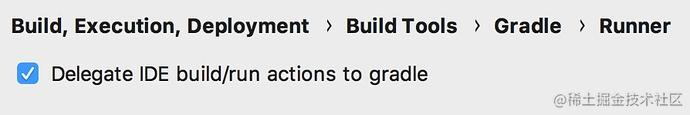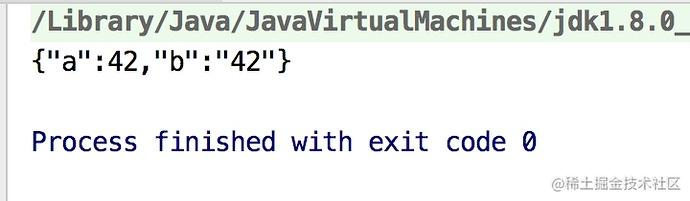## 来个嵌套的类型

``````data class User(val name: String, val birthDate: Date)

``````println(JSON.stringify(User("bennyhuo", Calendar.getInstance().apply { set(2000, 3, 1, 10, 24,0) }.time)))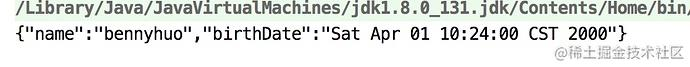``````@Serializer(forClass = Date::class)
object DateSerializer : KSerializer<Date> {
private val simpleDateFormat = SimpleDateFormat("yyyy-MM-dd HH:mm:ss")

override fun save(output: KOutput, obj: Date) {
output.writeStringValue(simpleDateFormat.format(obj))
}
}

``````@Serializable
data class User(val name: String,
@Serializable(with = DateSerializer::class) val birthDate: Date)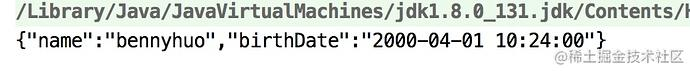## 更复杂一点儿的情况

``````@Serializable
class MyDate(var year: Int = 0, var month: Int = 0, var day: Int = 0,
var hour: Int = 0, var minute: Int = 0, var second: Int = 0){
... //省略 toString()
}

``````MyDate(2000, 3, 1, 10, 24, 0)

``````@Serializer(forClass = MyDate::class)
object MyDateSerializer : KSerializer<MyDate> {
private val jClassOfMyDate = MyDate::class.java

override fun load(input: KInput): MyDate {
val myDate = MyDate()
for (i in 0 until serialClassDesc.associatedFieldsCount) {
jClassOfMyDate.getDeclaredField(serialClassDesc.getElementName(i)).apply { isAccessible = true }.set(myDate, value)
}
return myDate
}

override fun save(output: KOutput, obj: MyDate) {
val arrayOutput = output.writeBegin(ArrayClassDesc, 0)
for (i in 0 until serialClassDesc.associatedFieldsCount) {
val value = jClassOfMyDate.getDeclaredField(serialClassDesc.getElementName(i)).apply { isAccessible = true }.get(obj) as Int
arrayOutput.writeIntElementValue(ArrayClassDesc, i + 1, value)
}
arrayOutput.writeEnd(ArrayClassDesc)
}
}

`save` 方法可以让我们在序列化 `MyDate` 的对象时按数组的形式输出，而 `load` 方法则用于反序列化。这段代码看上去有些古怪，不过不要感到害怕，一般情况下我们不会需要这样的代码。有了 `MyDateSerializer` 之后，我们需要注册它才可以使用，即：

``````val json = JSON(context = SerialContext().apply { registerSerializer(MyDate::class, MyDateSerializer) })
val result = json.stringify(MyDate(2000, 3, 1, 10, 24, 0)) //result = "[2000,3,1,10,24,0]"

``````@Serializable
data class User(val name: String,
@Serializable(with = DateSerializer::class) val birthDate: Date)

``````@Serializable(with = MyDateSerializer::class)
class MyDate(...){ ... }

## Gson 做不到的事儿

### ①构造方法默认值

``````com.google.code.gson:gson:\$version

``````@Serializable
data class Data(val a: Int, @Optional val b: String = "42")

``````val obj = JSON.parse<Data>("""{"a":42}""") // Data(a=42, b="42")
val objGson = gson.fromJson("""{"a":42}""", Data::class.java) //Data(a=42, b="?")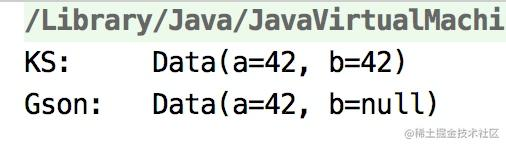### ②属性的初始化值

``````@Serializable
data class Data(val a: Int, @Optional val b: String = "42"){
@Optional
private val c: Long = 9

override fun toString(): String {
return "Data(a=\$a, b='\$b', c=\$c)"
}
}

``````val obj = JSON.parse<Data>("""{"a":42}""") // Data(a=42, b="42", c=?)
val objGson = gson.fromJson("""{"a":42}""", Data::class.java) //Data(a=42, b="null", c=?)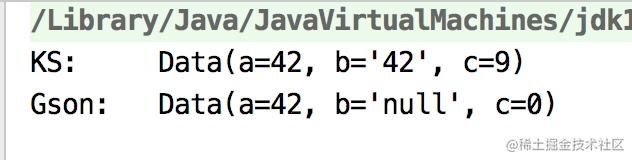### ③属性代理

``````@Serializable
data class Data(val a: Int, @Optional val b: String = "42"){
@Optional
private val c: Long = 9

@Transient val d by lazy { b.length }

override fun toString(): String {
return "Data(a=\$a, b='\$b', c=\$c, d=\$d)"
}
}

``````val obj = JSON.parse<Data>("""{"a":42}""") // Data(a=42, b="42", c=9, d=?)
val objGson = gson.fromJson("""{"a":42}""", Data::class.java) //Data(a=42, b=null, c=0, d=?)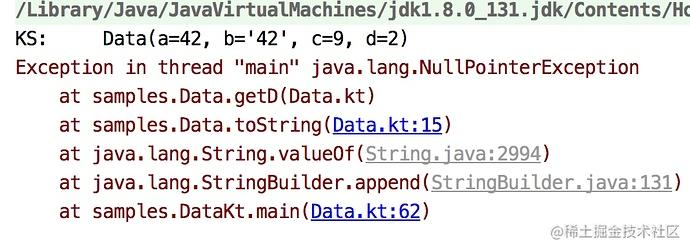``````private final Lazy d\$delegate;

## 小结

coding.imooc.com/class/232.h…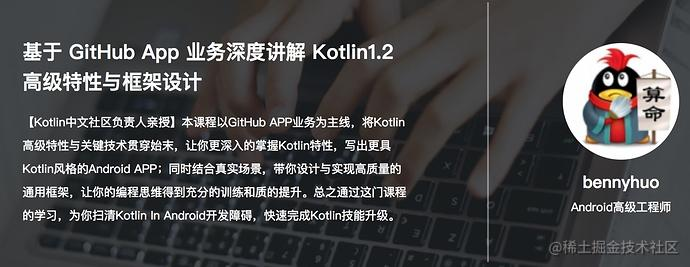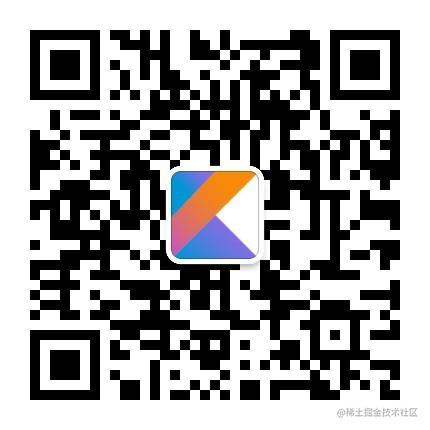Android